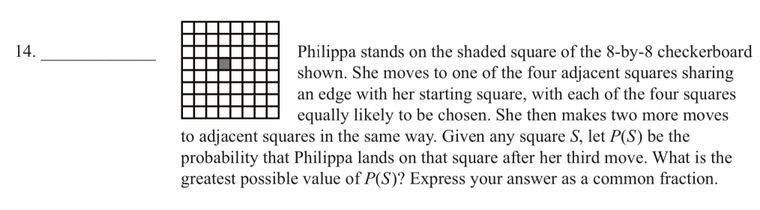# Mathcounts problems help

• Question 1.Why is the answer to this problem 9/64?

Question 2.
The diagonals of parallelogram ABCD intersect at E. Point F is the midpoint of segment BE and H is the midpoint of segment CE. What is the ratio of the area of quadrilateral AFHD to the area of the parallelogram? Express your answer as a common fraction.

Why isn’t the answer to this problem 1/2 ,but 9/16?
Question3.
Sam creates a six-digit positive integer by writing the digit 7 in the hundred-thousands place, and then tossing a fair coin five times. If the coin comes up heads, he writes a 7 for the next digit; if the coin comes up tails, he writes a 0 for the next digit. What is the probability that Sam’s number is divisible by 77? Express your answer as a common fraction.